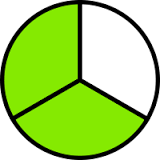# What is the meaning of 2/3?

## 3 Answers

Van Sy answered

Ratios are pairs of numbers and they are used to make comparisons.
A ratio compares two numbers using a fraction. Ratios can be written
three different ways:

(1) 2 to 3
(2) 2:3
(3) 2/3

They all mean the same thing.  Pretend I have a class of 25 students:
10 students are boys and 15 are girls.  I can compare number of boys
to girls using the ratio 10/15 (or 10:15; or 10 to 15). This ratio
means the same thing as saying that for every ten boys in my class, I
have 15 girls. You can "reduce" this fraction (remember, ratios are
comparisons using fractions) to 2/3 by dividing both numerator and
denominator by 5.

2 People thanked the writer.
Ancient Hippy answeredTom Jackson answered

2/3 is a ratio.

A ratio says how much of one thing there is compared to another thing.

2 People thanked the writer.

## Answer Question